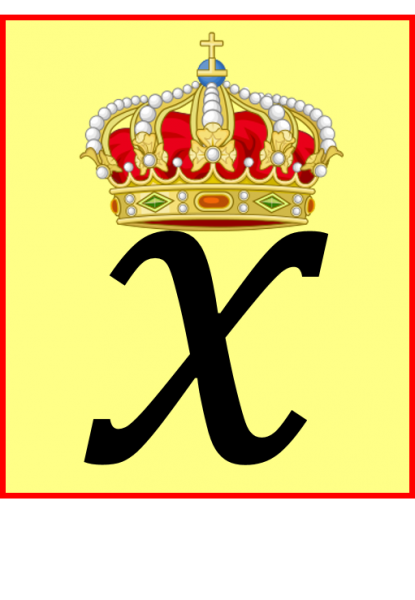07899 756585

# $x$ is the ideal letter to represent the unknown simply because the other letters are so busy doing other things! To demonstrate, here is a small (not exhaustive) collection of mathematical measures, physical quantities and units, using all upper and lowercase letters a to Z (with just two blanks – can you fill them?). Some will be familiar to primary School students; most if not all will be familiar by the end of an A-level mathematics course. Why not see if you can create your own algebra alphabet of mathematical letters first, before reading mine? Good luck!

## GOLD LEVEL: try the same exercise but using all the letters of the Greek alphabet e.g. $\pi =3.1415…$ (bonus points if you can use both the upper and lowercase Greeks!).

a, b, c: lengths of the sides of a triangle, trapezium e.g. Pythagoras $a^2+b^2=c^2$

A, B, C: the three angles in a triangle e.g. sine rule $\frac{a}{\sin(A)}=\frac{b}{\sin(B)}$

a: acceleration

A: Area e.g. area of circle $A=\pi r^2$

b: base e.g. area of triangle $A=\frac{b\times h}{2}$

B: denoting the Binomial distribution in statistics.

c: speed of light $c=3.0×10^{8}$ or prefix for “centi~” meaning $\times 10^{-2}$

C: circumference $C=\pi D$, or Celsius (the unit of temperature), or 100 in Roman Numerals.

d: distance.

D: Diameter of circle e.g. $C=\pi D$, or 500 in Roman Numerals.

e: Euler’s constant $e=2.718281828459045…$

E: Exterior angle of a polygon $E=\frac{360}{n}$ or Energy e.g. $E=mc^2$

f: a function $f(x)$, or no. of faces in Euler’s Polyhedron formula $f+v-e=2$

F: force e.g. Newton’s second law $F=ma$, denoting the F distribution in statistics (named after Fisher).

g: acceleration due to gravity g=9.81 (planet Earth) or g=1.625 (moon).

G: Newton’s Universal Gravitational Constant $G=6.674\times 10^{-11}$ e.g. Newton’s famous inverse square law $F=-\frac{GMm}{r^2}$

h: height.

H: [Help! Must be from School level Maths, not Chemistry or Physics].

i, j, k: unit vectors in the $x, y, z$ directions respectively.

i: used in complex numbers $i=\sqrt{-1}$

I: Impulse in mechanics $I=mv-mu$ or in physics the Moment of Inertia (the “rotational analogue of mass”).

j: alternative symbol for $i$ in complex numbers $j=\sqrt{-1}$

J: Joules (the unit of energy).

k: prefix for “kilo~” meaning $\times 10^3$

K: Kelvin (the S.I. unit of temperature – admittedly more physics than maths?).

l: length.

L: the number 50 in Roman Numerals.

m: mass e.g. $F=ma$ or metres, the unit of length.

M: another (larger) mass e.g. Newton’s inverse square law $F=-\frac{GMm}{r^2}$ or the prefix for “Mega~” meaning $\times 10^6$, or 1000 in Roman Numerals.

n: a unknown counting number e.g. “assume there are n people”, or “find the nth term”.

N: Newtons (the unit of force), or denoting a Normal distribution in statistics.

o, O: best avoided as so easily confused with 0 (zero).

p: sometimes used for momentum in mechanics $p=mv$

P: Pressure or Power, also commonly used as a name for a point in the plane.

q: [help?!]

Q: another point in the plane; electrical charge $Q=It$

r: radius e.g. area of a circle $A=π r^2$

R: electrical resistance $V=IR$

s: displacement in constant acceleration formulae e.g. $s=ut+\frac{1}{2}at^2$; seconds (S.I. unit of time); the sample standard deviation in statistics $s=\sqrt{\frac {\Sigma (x-\bar{x})^2}{n-1}}$.

S: sum e.g sum of n terms of a geometric series $S_{n}=\frac{a(1-r^n)}{1-r}$

t: time, or denoting the t distribution in statistics.

T: Temperature, or an unknown value of t, the time.

u:  the initial velocity.

U: denoting the Uniform Distribution in statistics.

v: (final) velocity e.g. Kinetic Energy $E_{k}=\frac{1}{2}mv^2$

V: volume e.g. volume of sphere $V=\frac{4}{3}π r^3$, or 5 in Roman Numerals.

w: width e.g. area of rectangle $A=l× w$

W: Watts (the unit of power), Weight $W=mg$, Work Done $W=Fs$

x: free to stand for a general unknown number!!

X: free to use as a general random variable in statistics, or 10 in Roman Numerals.

y: likewise, free to stand for a second unknown number.

Y: free to use as a second random variable in statistics.

z: free to represent a third unknown number. Typically used for an unknown complex number.

Z: in statistics, a random variable with a standardised Normal distribution.

##### NOTIFY ME OF NEW POSTS BY EMAIL:

[mc4wp_form id=”399″]